# Notes on TeX and LaTeX

What follows is a set of notes and examples, in no particular order, on how to do various things, some simple, some a little bit twisted, with TEX and LATEX.

## Contributing

If I've made a mistake somewhere, or haven't covered something that you think should be covered, please mail me about it.

Contributors:

## Resources

Recommended reading: The (Not So) Short Introduction to LATEX 2e - many systems will have it in texmf/doc/latex/general/lshort.dvi.

## The template LaTeX Document

\documentclass[a4paper]{article}
\begin{document}

% write stuff here

\end{document}


## Arithmetic in a macro

\def\add#1#2{
\newcount\tmp
\tmp=#1
\number\tmp
}


\add{1}{2} produces 3. As well as \advance, you can use \divide and \multiply, but be aware that these are all integer operations.

## To insert the contents of another file

\input part1


TEX will guess the .tex suffix, so the above is equivalent to:

\input part1.tex


The \input command inserts the contents of the specified file at the current location. It can be used to split a large .tex file into more manageable chunks.

## Variable vertical gaps

Line
\\[6pt]
Another line


The size of the gap can be given in any units. It can also be negative.

## Smaller margins

Before \begin{document}, add something like:

\usepackage[nohead,margin=2cm,includefoot]{geometry}


The geometry package can be used to manipulate margins in many interesting ways. Be sure to read the documentation that comes with it. Also, make sure you have a sufficiently recent version of the package - you can download the latest one from CTAN and keep the geometry.cfg and geometry.sty files in the same directory as your .tex source.

There is also the slightly more standard fullpage package that will set 1-inch margins:

\usepackage{fullpage}


Or 1.5cm margins:

\usepackage[cm]{fullpage}


## Crash course in tables

To produce a table like: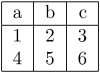Use something like the following:

\begin{tabular}{|l|c|r|}
\hline
a & b & c\\
\hline
1 & 2 & 3\\
4 & 5 & 6\\
\hline
\end{tabular}


Left, centered, and right column alignments are specified as l, c, and r, respectively. The bars (|) between column alignment letters become vertical borders in the table. Horizontal borders are created using \hline.

Cells are separated with ampersands (&) and rows are terminated using the line break (\\).

## Using EPS figures

Before \begin{document}, add \usepackage{graphicx}.
Then you can insert a figure using:

\begin{figure}[h]
\includegraphics{figure.eps}
\end{figure}


h stands for "place the figure here." The other possible placements are t and b for top and bottom, respectively.

To scale the figure:

\begin{figure}[h]
\includegraphics[scale=0.5]{figure.eps}
\end{figure}


To center the figure:

\begin{figure}[h]
\centering
\includegraphics{figure.eps}
\end{figure}


\begin{figure}[h]
\includegraphics{figure.eps}
\caption{Representation of stuff}
\end{figure}


To put a border around the figure:

\begin{figure}[h]
\fbox{ \includegraphics{figure.eps} }
\end{figure}


To be able to reference the figure in text afterwards:

\begin{figure}[h]
\includegraphics{figure.eps}
\caption{Representation of stuff}
\label{fig:stuff}
\end{figure}



Note that you must have a \caption. You will also have to process the document twice to generate correct references.

## Basic formatting

\textbf{bold}
\textit{italic}
\underline{underlined}
\texttt{typewriter, monospace}
\textsf{sans serif}
\textsc{small caps}


Text is justified by default. To force left alignment:

\begin{flushleft}
Text goes here.
\end{flushleft}


Text sizes, from smallest to largest:

{\tiny         text}
{\scriptsize   text}
{\footnotesize text}
{\small        text}
{\normalsize   text}
{\large        text}
{\Large        text}
{\LARGE        text}
{\huge         text}
{\Huge         text}


## Page breaks

In LATEX:

\newpage


In plain TEX:

\eject


You may wish to use \vfil immediately before \eject or TEX will try to stretch the contents vertically to fill the whole page.

## Columns

After specifying the \documentclass:

\usepackage{multicol}
\setlength{\columnsep}{1.5pc}  % space between cols
\setlength\columnseprule{.4pt} % line between cols


Then, in the body of the document:

\begin{multicols}{2}
Stuff
\columnbreak
More stuff.
\end{multicols}


## Duplex printing

Make a file, for example duplex.pro that holds the following line of PostScript:

<< /Duplex true >> setpagedevice


Then, process your DVI into PS using:

dvips -h duplex.pro -o out.ps in.dvi


## Hiding the page number

To suppress all page numbers in the entire document:

\usepackage{nopageno}


Alternately, page numbers can be suppressed on a page-by-page basis by using:

\thispagestyle{empty}


For plain TEX, use:

\nopagenumbers


## Hybrid formulae

To produce a formula like: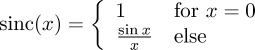Use something like the following:

\begin{displaymath}
\textrm{sinc}(x) = \left\{
\begin{array}{ll}
1                & \textrm{for } x=0 \\
\frac{\sin x}{x} & \textrm{else}
\end{array}
\right.
\end{displaymath}


## Un-numbered sections

To suppress the number before a section title, use:

\section*{No number due to asterisk}


## De-indenting Paragraphs

A paragraph indent can be suppressed by prefixing it with \noindent. To use vertical spacing instead of paragraph indents across the entire document, add the following before \begin{document}:

\setlength{\parindent}{0pt}
\setlength{\parskip}{\baselineskip}


Or, use the parskip package:

\usepackage{parskip}


## Landscape

\usepackage{lscape}, then use \begin{landscape}...\end{landscape} for parts of the document that are desired in landscape.

## Binomial coefficient / nCr / choose

To get a pretty binomial coefficient like: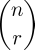Use: n \choose r in math mode.

## Arbitrary font size

To set the font size using LATEX2e:

Here is some text in a
{\fontsize{14pt}{16pt}\selectfont different size}.


The first parameter (14pt) specifies the font size, the second parameter sets the baseline skip.

## Really large fonts

When using the Computer Modern typeface, calls to \fontsize will round the specified size to one of a number of presets. For example:

{\fontsize{60pt}{60pt}\selectfont BIG}


Results in:

LaTeX Font Warning: Font shape OT1/cmr/m/n' in size <60> not available, size <24.88> substituted.

To get text at any arbitrary size, use a Type 1 font. For example, Adobe Times (the ptm family):

{\fontfamily{ptm}\fontsize{60pt}{60pt}\selectfont BIG}


## Vertically centering a page

\vspace*{\fill}
...contents...
\vspace*{\fill}


## Changing itemize spacing

In the itemize environment, the spacing between items and paragraphs is determined by the lengths \itemsep and \parskip. Unfortunately, these lengths are reset upon entering the itemize environment, so setting them outside of the environment has no effect, and the solution becomes a little bit hacky.

Hijack the \begin macro, so that \begin{itemize} will enter the itemize environment, then set \itemsep and \parskip to the desired values:

\makeatletter
\let\origbegin=\begin
\def\begin#1{
\origbegin{#1}
\def\@a{#1}
\def\@b{itemize}
\ifx\@a\@b
\fi
}
\makeatother


Thanks to Iman S. H. Suyoto for suggesting the use of @.

## Color

Color is represented using a DVI special. When using LATEX, there is a color package that makes this painless:

\usepackage{color}
...
% "some colored text"
some \textcolor{red}{colored} text


In plain TEX:

some \special{color push rgb 1 0 0}colored\special{color pop} text


You can specify color as:
color push rgb (r) (g) (b)
color push cmyk (c) (m) (y) (k)
color push gray (g)
or by name, e.g.
color push Black

If specifying by value, the values are floats between 0 and 1.

## Boxes, Baselines, Height and Depth

TEX works by arranging boxes and glue. Each character is a box. Character boxes are merged into words. Word boxes, and inter-word spacing (which is glue), is merged into lines. Each line is a box. Finally, a page is a box containing lines, diagrams, and other such boxes.

Here is \hbox{hg} with the box and baseline drawn in: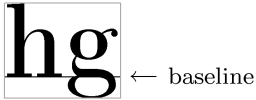The baseline is the line that all characters "sit" on. A line on the page will have all elements lined up so that they sit on the same baseline.

The height of a box is a measure of how far it extends above the baseline.

Some characters dip below the baseline. The extent of this is called the depth of the box.

## \vbox and \vtop

A \vbox is a box that has contents in vertical mode.

\vbox{hello} would produce a box as wide as the page, containing the word "hello"

To limit the width to the minimum needed for the contents, we would wrap the contents in an \hbox. i.e.: \vbox{\hbox{hello}}

A \vbox could contain multiple "lines" and still be used as an element in horizontal mode. Remember that TEX arranges all elements on the same baseline. The baseline of a \vbox is the baseline of its bottom element.

A \vtop is the same as a \vbox except its baseline is the baseline of the topmost element.

Example:

\def\vhb#1{\vbox{\hbox{#1}}}

A vbox:
\vbox{\vhb{eight}\vhb{gnat}}
and a vtop:
\vtop{\vhb{eight}\vhb{gnat}}
on one line.
`

With visible boxes and baselines: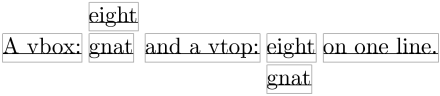Path: home > stuff > Notes on TeX and LaTeX